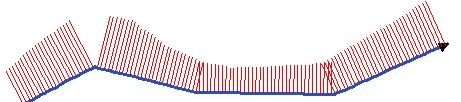Go to ET GeoWizards 12.x User Guide

Create Station Lines

Go to ToolBox Implementation

Creates equally spaced lines along the polylines from the input dataset. The station lines are single segmented polylines perpendicular to the input polylines (at the location of the station).

Inputs:

• A polyline feature layer
• Distance between stations
• Side of the station lines - three options are available
• Both - the middle of the station lines will intersect the original polylines
• Left - station lines will be located on the left side of the polylines
• Right - station lines will be located on the right side of the polylines
• Length of the station lines can be specified
• Constant - all the station lines will have the same user specified length
• M Values - the M value of the input polylines (at the location of the station) will be used for length of the station lines. The input polylines must have M values.
• Z  Values - the Z value of the input polylines (at the location of the station) will be used for length of the station lines. The input polylines must have Z values.

Outputs:

• New Polyline feature class with single segmented polylines perpendicular to the input polylines, distributed along the input polylines based on the user selected options.
• The attributes of the original polylines are preserved
• The following fields are added to the point attribute table
• [ET_ID] - the FID of original polylines.
• [ET_Angle] - the angle of the polyline at the station.
• [ET_Station] - the distance from the start point of the polyline to the station line
• [ET_Length] - the length of the station line

Notes:

• The distance is measured in the units of the spatial reference of the input dataset
• The output spatial reference is the one of the input polyline dataset
Examples:Side = "Both" Step = 200 meters Constant Length = 100 metersSide = "Right" Step = 100 meters Constant Length = 100 metersSide = "Left" Step = 20 meters Length from Z values

Command line syntax

ET_GPStationLines<input_dataset> <out_feature class> <station_distance> <Both | Left | Right> <Constant | M Values | Z Values> {lines_length}

Parameters

Expression Explanation
<input_dataset> A Polyline or Polygon feature class or feature layer
<out_feature class> A String - the full name of the output feature class (A feature class with the same full name should not exist)
<station_distance> A Double representing the distance (in the units of the spatial refere4nce of the input feature class) between the station lines along the input polylines
<Both | Left | Right> A String representing the side of the station lines in relation to the original polylines.
<Constant | M Values | Z Values> A String indicating where the length of the station lines will be taken from
{lines_length} A Double representing the length (in the units of the spatial refere4nce of the input feature class) of the station lines. Used only with "Constant" option.

Scripting syntax

ET_GPStationLines(input_dataset, out_feature class,station_distance, side, length_from, lines_length)

See the explanations above:
<> - required parameter
{} - optional parameter